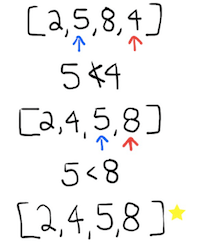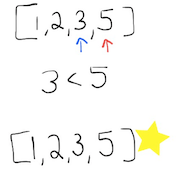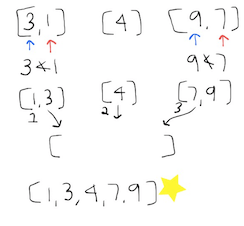# Sorting Algorithms Conceptually

If you’re interested in computer science, or just different ways to sort a list of numbers, knowing some basic sorting algorithms will be very helpful for you. OR you may be interested in folk dancing… then this is also the post for you, because all of the different types of sorting titles are linked to a video where it is explained through folk dancing!

First you should know what an array is. An array is a data structure that holds a group of elements that are all the same data type (all letters, all numbers, etc.). If this concept throws you off, every time I say array, just replace it in your head with the word list(like a grocery list). I will be sorting number arrays.

WE WILL BE SORTING FROM SMALLEST TO LARGEST IN EVERY EXAMPLE

Selection sortThe blue arrow is pointing to what we are looking at, and the red arrow is what we are comparing it to. There are brackets around the numbers to show that this is an array.
1. You point to the first element in the array, and then the second element, and compare the two. Because the blue number is larger than the red number we incrament(increaste by one) and turn the current red number into the new blue number. The old blue number is no longer being pointed to.
2. We keep comparing 2 elements in the array, and stop pointing to the larger number of the two. We then keep comparing the smaller number to the next elements in the array until we prove that it is the smallest, and then it is put at the front of the array where it will stay.
3. Keep going through the process for the length of the array, incrementing(increasing by 1)the position that you put the current smallest number in. The last few elements don’t take too long because they have fewer things to be compared with.

Bubble SortThe blue arrow is pointing to what we are looking at, and the red arrow is what we are comparing it to. There are brackets around the numbers to show that this is an array.
1. Point to the first element and compare it to the second element in the array.
2. If the first is smaller, increment what element in the array your blue number is. If the your blue number is larger than your red number you swap the elements. Then we compare the next two elements(so on and so forth).
3. The array will not be sorted after this.
4. Once you have parsed through the end of the array, then go through the array again and do the same thing. At each pass, we aren’t finding the largest element, but putting it one position closer to where its final position is.
5. If you pass through the array and do not make a swap, that is how you know that you have finished and your array is sorted.The blue arrow is pointing to what we are looking at, and the red arrow is what we are comparing it to. There are brackets around the numbers to show that this is an array.
1. You start this sorting process by splitting the array into two arrays. Make them equal sizes if possible.
2. Once you split the original array, then split the individual sides until they are broken down into arrays with one element in them. An array with one element is technically already sorted. Then you merge it (compare and put in order) with the closest singular array.
3. Then repeat until we have one of the original sides sorted. We repeat this processes for the other side.
4. You then merge the two sorted and split arrays by comparing the first elements and putting the smaller of the two into a new ,third, array. You increment(increase by one) through the two sorted arrays as you add elements into the new array. You compare as you go until there are no more elements in either of the two sorted arrays.
5. *If the two elements are equal to each other you write a special line of code saying which side to take from in this condition.

Quick SortThe red arrow is symbolic of your pivot number, and the blue arrow is the number you are currently comparing to the pivot number. There are brackets around the numbers to show that this is an array.
1. You have your unsorted (shuffled) array, and then randomly select a number in your array to be your “pivot” number.
2. Now you split the array into three new arrays. The first array is everything that is smaller than the pivot number, the second array is the pivot itself, and the third array is everything larger than the pivot number.
3. Then you sort the first array by doing quick sort again (randomly picking a pivot number, and then splitting the array into everything larger and smaller than the pivot) and keep going until the smaller than the pivot array and larger than the pivot array only contain one number.
4. You then make a new array, and stick your original first array, that is now sorted, in front of your original pivot, and sort the third array(the same way as the first) and stick it after the pivot. Since the pivot is only one number is it technically already sorted.
5. We now have a sorted array.

Let me know in the comments if this helped you better understand sorting algorithms!

Claps on this post are very appreciated